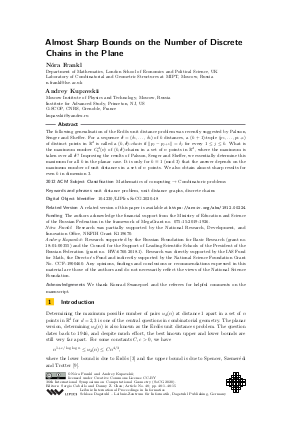Document# Almost Sharp Bounds on the Number of Discrete Chains in the Plane

### Authors Nóra Frankl, Andrey Kupavskii## File

LIPIcs.SoCG.2020.48.pdf
• Filesize: 0.54 MB
• 15 pages

## Cite As

Nóra Frankl and Andrey Kupavskii. Almost Sharp Bounds on the Number of Discrete Chains in the Plane. In 36th International Symposium on Computational Geometry (SoCG 2020). Leibniz International Proceedings in Informatics (LIPIcs), Volume 164, pp. 48:1-48:15, Schloss Dagstuhl - Leibniz-Zentrum für Informatik (2020)
https://doi.org/10.4230/LIPIcs.SoCG.2020.48

## Abstract

The following generalisation of the Erdős unit distance problem was recently suggested by Palsson, Senger and Sheffer. For a sequence δ=(δ₁,… ,δ_k) of k distances, a (k+1)-tuple (p₁,… ,p_{k+1}) of distinct points in ℝ^d is called a (k,δ)-chain if ‖p_j-p_{j+1}‖ = δ_j for every 1 ≤ j ≤ k. What is the maximum number C_k^d(n) of (k,δ)-chains in a set of n points in ℝ^d, where the maximum is taken over all δ? Improving the results of Palsson, Senger and Sheffer, we essentially determine this maximum for all k in the planar case. It is only for k ≡ 1 (mod 3) that the answer depends on the maximum number of unit distances in a set of n points. We also obtain almost sharp results for even k in dimension 3.

## Subject Classification

##### ACM Subject Classification
• Mathematics of computing → Combinatoric problems
##### Keywords
• unit distance problem
• unit distance graphs
• discrete chains

## Metrics

• Access Statistics
• Total Accesses (updated on a weekly basis)
0

## References

1. P. K. Agarwal, E. Nevo, J. Pach, R. Pinchasi, M. Sharir, and S. Smorodinsky. Lenses in arrangements of pseudo-circles and their applications. J. ACM, 51(2):139-186, 2004.2. B. Aronov and M. Sharir. Cutting circles into pseudo-segments and improved bounds for incidences. Discrete Comput. Geom., 28(4):475-490, 2002.3. P. Erdős. On sets of distances of n points. Amer. Math. Monthly, 53(5):248-250, 1946.4. P. Erdős. On sets of distances of n points in euclidean space. Magyar Tud. Akad. Mat. Kutato Int. Közl., 5:165-169, 1960.5. H. Kaplan, J. Matoušek, Z. Safernová, and M. Sharir. Unit distances in three dimensions. Comb. Probab. Comput., 21(4):597-610, 2012.6. A. Marcus and G. Tardos. Intersection reverse sequences and geometric applications. J. Combin. Theory Ser. A, 113(4):675-691, 2006.7. J. Pach and M. Sharir. Geometric incidences. In Towards a theory of geometric graphs, volume 342 of Contemp. Math., pages 185-223. Amer. Math. Soc., Providence, RI, 2004.8. E. A. Palsson, S. Senger, and A. Sheffer. On the number of discrete chains, 2019. URL: http://arxiv.org/abs/1902.08259.
9. J. Spencer, E. Szemerédi, and W. T Trotter. Unit distances in the Euclidean plane. In Graph theory and combinatorics, pages 294-304. Academic Press, 1984.10. J. Zahl. An improved bound on the number of point-surface incidences in three dimensions. Contrib. Discrete Math., 8(1):100-121, 2013.11. J. Zahl. Breaking the 3/2 barrier for unit distances in three dimensions. Int. Math. Res. Notices, 2019(20):6235-6284, 2019.X

Feedback for Dagstuhl Publishing